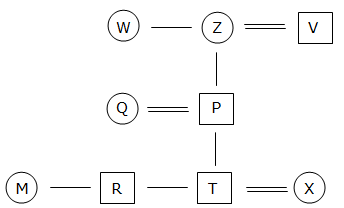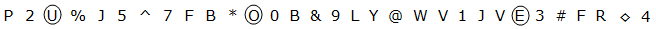# Reasoning  Miscellaneous – 1

D.1-3) Study the following information carefully and answer the given questions.

Q is the mother of M who is the sister of R and T. R is the son of P who is the child of Z. W is the sister of Z. V is the father in law of Q who have more than one son. X is married to T.

1) How is X related to Q?

a) Sister-in-law

b) Daughter-in-law

c) Son-in-law

d) Can’t be determined

e) None of these

b) Daughter-in-law2) If S is the sister of P then how is Q related to S?

a) Aunt

b) Wife

c) Sister-in-law

d) Daughter

e) None of these

c) Sister-in-law3) How is P related to W?

a) Daughter

b) Son

c) Niece

d) Nephew

e) None of these

d) NephewD.4-6) Study the following information and answer the given questions.

Q 2 V % K 5 ^ 7 E A * P 0 C & 9 M Z @ X U 1 I W F 3 # G S ◊ 4

4) How many such pairs are there in the arrangement in each of which vowels are immediately preceded by a number and immediately followed by a symbol?

a) One

b) Two

c) More than three

d) None of these

e) None

e) None

5) If in the above sequence all the symbols are dropped, then which of the following element is 5th to the right of 9th from the right end?

a) F

b) 1

c) 3

d) W

e) K

c) 3

After dropping all the symbols from the arrangement

9th from the left end –U

5th to the right of U-3

6) Four of the following five are alike in a certain way based on the above arrangement and hence form a group. Which of the following does not belong to that group?

a) K ^ V

b) * 0 E

c) @ U M

d) X 1 Z

e) F # 4

e) F # 4

all the others are followed same pattern

(i.e) K ^ V

=> K is middle element

=> ^ is second element from right side of K

=> V is second element from left side of K

7) In the following series what should come in place of question mark?

2%5 7AP &M@ ?

a) 1W3

b) IF#

c) @M&

d) F#S

e) 3G◊

b) IF#

2 % 5 7 A P & M @ IF#

8) If all the vowels are coded as its next alphabetical letter, and all the consonants are coded as its previous alphabetical letter then how many vowels are there in the arrangement?

a) One

b) Two

c) Four

d) Three

e) None